$1.54/Each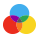Colors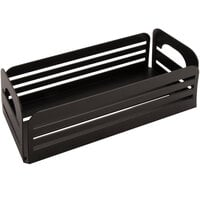$23.31/EachColors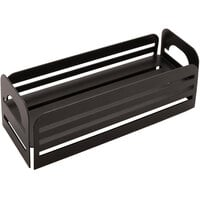$23.38/EachColors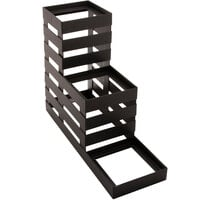From$45.32/Each

23+
$46.99$45.32Colors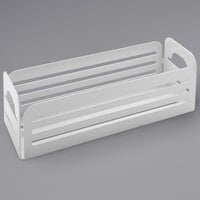$23.31/EachColors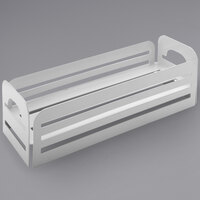$23.38/EachColors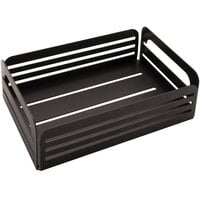$29.11/Each$29.11/Each

$10.68/Each$10.68/Each

$38.43/Each$38.43/Each

From $45.32/Each 23+$46.99\$45.32